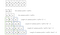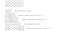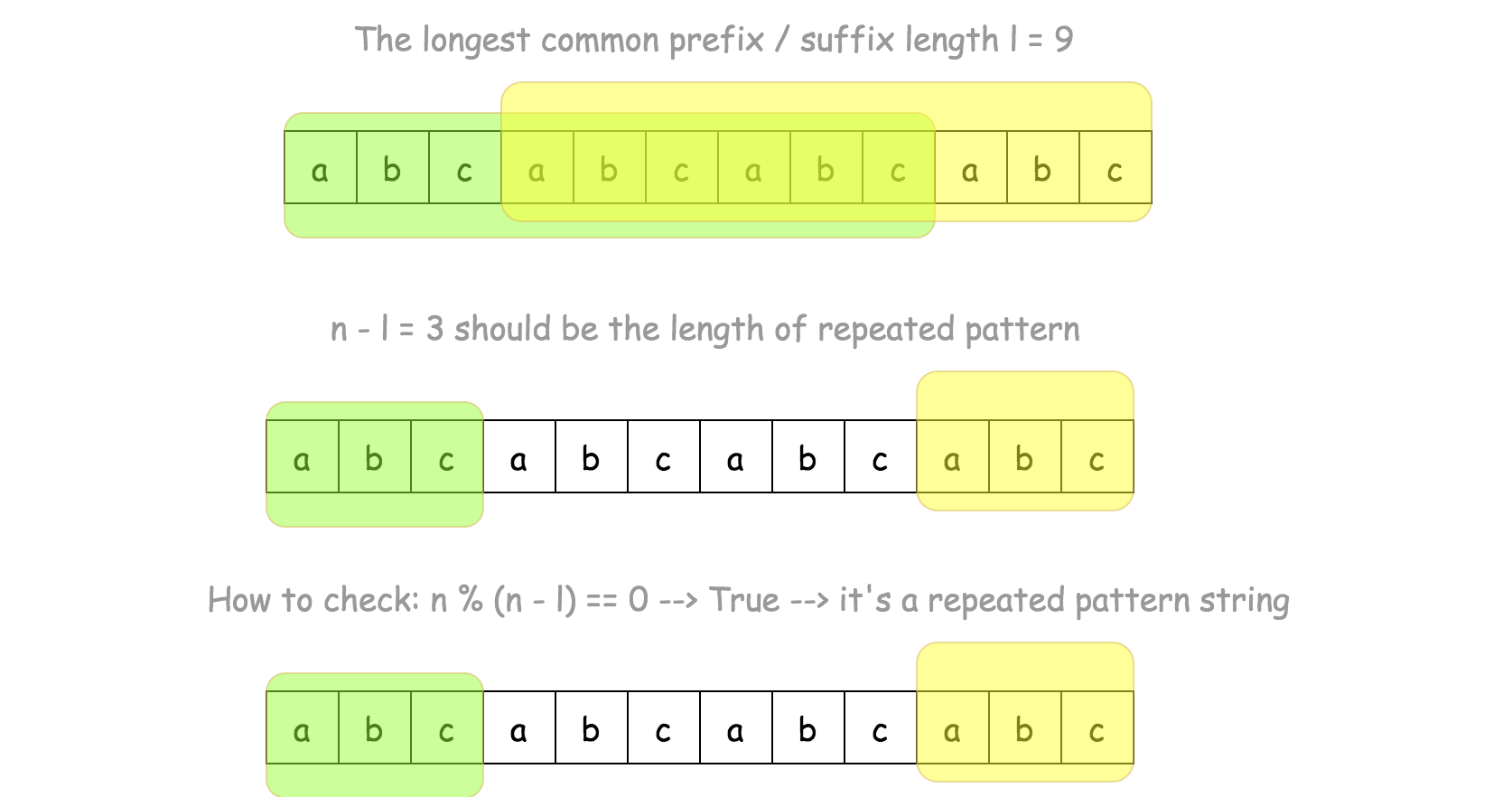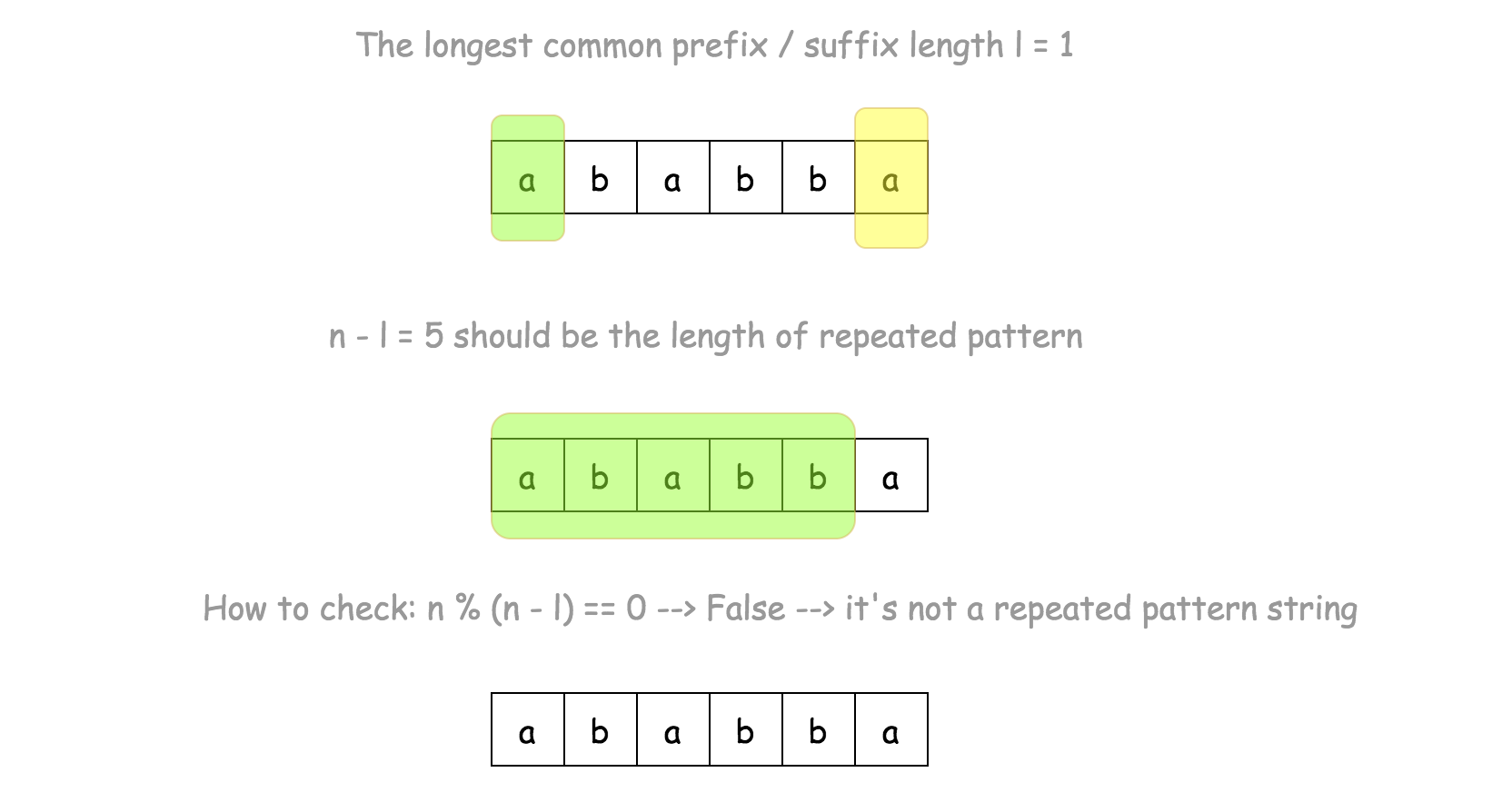# Repeated Substring Pattern: Leetcode tough KMP and Robin Karp Algorithm

https://leetcode.com/problems/repeated-substring-pattern/

Easy But tough for me as I do not know two of the algorithms

# 2. Divisors + Rabin-Karp

Given a non-empty string check if it can be constructed by taking a substring of it and appending multiple copies of the substring together. You may assume the given string consists of lowercase English letters only and its length will not exceed 10000.

Example 1:

`Input: "abab"Output: TrueExplanation: It's the substring "ab" twice.`

Example 2:

`Input: "aba"Output: False`

Example 3:

`Input: "abcabcabcabc"Output: TrueExplanation: It's the substring "abc" four times. (And the substring "abcabc" twice.)`

Solution:

My intuition: We use the last character of the string. This last character is also the last character of the substring which we suspect is being repeated. So we search for this last character from the front of the string. When we find it, we use the substring from 0 to that index as the substring that we suspect ( “sups”). Then we loop the string to ensure the rest of the string is composed of only suspected string.

`class Solution:    def repeatedSubstringPattern(self, s: str) -> bool:        # 11:00 am 11:14 am                if not s: return True        if len(s) == 1: return False                # find the index of the last character:        susp = ""        for i, x in enumerate(s):            if s[-1] == x:                susp = s[:i+1]                break        if susp == "":            return False        i = 0        while i < len(s):            if s[i:i+len(susp)] != susp:                return False            else:                i += len(susp)                        return True`

The solution part of this problem makes use of Approach 4: Knuth-Morris-Pratt Algorithm (KMP) and makes use of Approach 3: Find Divisors + Rabin-Karp. If you need to study these two algorithms and see where these are used. may be this is a good starting point : https://leetcode.com/problems/repeated-substring-pattern/solution/

# Solution

## Overview

The problem could be solved in many ways. Easy approaches have O(N2)O(N2)time complexity, though one could improve it by using one of string searching algorithms.

## Approach 3: Find Divisors + Rabin-Karp

Rabin-Karp

Rabin-Karp is a linear-time O(N) string searching algorithm:

• Move a sliding window of length LL along the string of length NN.
• Check hash of the string in the sliding window.

In some situations, one has to implement a particular hash algorithm to fit in a linear time, for example, we used rolling hash algorithm for the problem Longest Duplicate Substring.

For the current problem the standard `hash` / `hashCode` is enough because the idea is to check only lengths `L`, which are divisors of `N`. This way we're not sliding, we're jumping:

• the first string is `0..L`
• the second string is `L..2L`
• the last string is `N - L..N`

To copy characters in sliding window takes time `L`, to compute hash - time `L` as well. In total, there are `N / L` substrings, that makes it all work in a linear time O(N)O(N).

Find divisors

Now the only problem is to find divisors of `N`. Let's iterate to the square root of `N`, and for each identified divisor `i` calculate the paired divisor `N / i`.

Algorithm

Side note. The good practice is to verify the equality of two substrings after the hash match. This logic is not hard to add, and it could bring you kudos during the interview.

Implementation

`class Solution:    def repeatedSubstringPattern(self, s: str) -> bool:                n = len(s)        if n < 2:            return False        if n == 2:            return s == s                    for i in range(int(n**0.5), 0, -1):            if n % i == 0:                divisors = [i]                if i != 1:                    divisors.append(n // i)                for l in divisors:                    first_hash = curr_hash = hash(s[:l])                    start = l                    while start != n and curr_hash == first_hash:                        curr_hash = hash(s[start:start + l])                        start += l                    if start == n and curr_hash == first_hash:                        return True                        return False`

Complexity Analysis

• Time complexity: up to O(N * sqrt(N)). O(sqrt(N)) to compute all divisors and O(N) for each divisor “verification”. That’s an upper-bound estimation because divisor function grows slower than sqrt(N).
• Space complexity: up to O(sqrt(N)) to keep a copy of each substring during the hash computation.

## Approach 4: Knuth-Morris-Pratt Algorithm (KMP)

Algorithm : https://www.youtube.com/watch?v=GTJr8OvyEVQ and the Longest prefix array algorithm is https://www.youtube.com/watch?v=KG44VoDtsAA

Code is well explained in https://www.youtube.com/watch?v=4jY57Ehc14Y

Lookup Table :

# Rabin-Karp is the best fit for the multiple pattern search, whereas KMP is typically used for the single pattern search.

The key to KMP is the partial match table (PMT), often called lookup table(LP, or failure function table or longest prefix array. It stores the length of the longest prefix that is also a suffix.

Here are two examplesFirst use case of a pattern2nd use case of the pattern

How to Get an Answer

Once we have a lookup table, we know the length `l` of common prefix/suffix for the input string: `l = dp[n - 1]`.

That means that `n - l` should the length of the repeated sequence. To confirm that, one should verify if `n - l` is a divisor of `n`.Repeated pattern stringNot a repeated pattern string.

Algorithm

Implementation

`class Solution:    def repeatedSubstringPattern(self, s: str) -> bool:                n = len(s)        dp =  * n        # Construct partial match table (lookup table).        # It stores the length of the proper prefix that is also a proper suffix.        # ex. ababa --> [0, 0, 1, 2, 1]        # ab --> the length of common prefix / suffix = 0        # aba --> the length of common prefix / suffix = 1        # abab --> the length of common prefix / suffix = 2        # ababa --> the length of common prefix / suffix = 1        for i in range(1, n):            j = dp[i - 1]            while j > 0 and s[i] != s[j]:                j = dp[j - 1]            if s[i] == s[j]:                j += 1            dp[i] = j        l = dp[n - 1]        # check if it's repeated pattern string        return l != 0 and n % (n - l) == 0`

Based on the youtube videos My solution is :

`class Solution:    def repeatedSubstringPattern(self, s: str) -> bool:        # 11:00 am 11:14 am        # took me like 3 hours to learn the algorithm first and then see how can I apply it here        longest_prefix_array =  * len(s)                # the substring length will be the value of longest_prefix_array[-1]        # following algorihtm https://www.youtube.com/watch?v=KG44VoDtsAA        j = 0        i = 1        while i < len(s):            if s[j] == s[i]:                longest_prefix_array[i] = j + 1                j += 1                i += 1            else:                if j != 0:                    j = longest_prefix_array[j -1]                else:                    longest_prefix_array[i] = 0                    i += 1        # length of the substring is                     l = longest_prefix_array[-1]        print("substring" , s[0:l])        n = len(s)                # check if it's repeated pattern string        # if this length is nonzero and n - l is a divisor of n        print(len(s) % (len(s) - l) == 0)                return l != 0 and n % (n - l) == 0`

Complexity Analysis

• Time complexity: O(N). During the execution, `j` could be decreased at most N times and then increased at most N times, that makes overall execution time to be linear O(N).
• Space complexity: O(N) to keep the lookup table.

…………………………………………………………………………………………………

# Other approaches :

## Approach 1: Regex

To use regex during the interviews is like to use built-in functions, the community has no single opinion about it yet, and it’s a sort of risk.

Implementation

`import reclass Solution:    def repeatedSubstringPattern(self, s: str) -> bool:        pattern = re.compile(r'^(.+)\1+\$')        return pattern.match(s)`

Complexity Analysis

• Time complexity: O(N2) or O(N-suqare) because we use greedy regex pattern. Once we have a `+`, the pattern is greedy.
• The difference between the greedy and the non-greedy match is the following:
• the non-greedy match will try to match as few repetitions of the quantified pattern as possible.
• the greedy match will try to match as many repetitions as possible.
• The worst-case situation here is to check all possible pattern lengths from `N` to `1` that would result in O(N-square) time complexity.
• Space complexity: O(1). We don’t use any additional data structures, and everything depends on internal regex implementation, which is evolving quite fast nowadays. If you’re interested to dig depeer, here is a famous article by Russ Cox which inspired a lot of discussions and code changes in Python community.

## Approach 2: Concatenation

Repeated pattern string looks like `PatternPattern`, and the others like `Pattern1Pattern2`.

Let’s double the input string:

`PatternPattern` --> `PatternPatternPatternPattern`

`Pattern1Pattern2` --> `Pattern1Pattern2Pattern1Pattern2`

Now let’s cut the first and the last characters in the doubled string:

`PatternPattern` --> `*atternPatternPatternPatter*`

`Pattern1Pattern2` --> `*attern1Pattern2Pattern1Pattern*`

It’s quite evident that if the new string contains the input string, the input string is a repeated pattern string.

Implementation

`class Solution:    def repeatedSubstringPattern(self, s: str) -> bool:        return s in (s + s)[1: -1]`

Complexity Analysis

• Time complexity: O(N-square) because of the way `in` and `contains` are implemented.
• Space complexity: O(N), the space is implicitly used to keep `s + s`string.

## More from Technical Interviews Preparation

Currently preparing for interviews